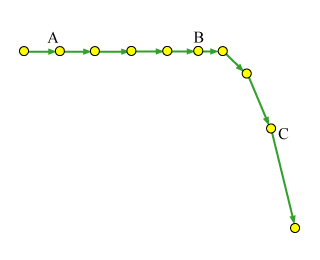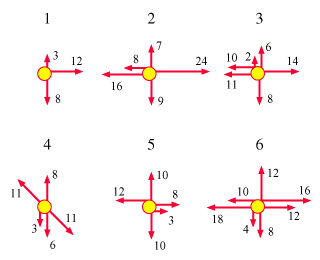# Problem: To understand the meaning and the basic applications of Newton's 1st and 2nd laws.In this problem, you are given a diagram (Figure 1) representing the motion of an object--a motion diagram. The dots represent the object's position at moments separated by equal intervals of time. The dots are connected by arrows representing the object's average velocity during the corresponding time interval.Your goal is to use this motion diagram to determine the direction of the net force acting on the object. You will then determine which force diagrams and which situations may correspond to such a motion.Figure 1Figure 2Part FWhich of these diagrams may possibly correspond to the situation at point C on the motion diagram?Type, in increasing order, the numbers corresponding to the correct diagrams. Do not use commas. For instance, if you think that only diagrams 3 and 4 are correct, type 34.

###### FREE Expert Solution

In this problem, we'll determine the net force from the velocity-time graph and compare it with the net force from the force diagrams.

Newton's second law:

$\overline{){\mathbf{\Sigma }}{\mathbf{F}}{\mathbf{=}}{\mathbf{m}}{\mathbf{a}}}$

Newton's second law implies that the acceleration is in the direction of the net force.

87% (411 ratings)###### Problem Details

To understand the meaning and the basic applications of Newton's 1st and 2nd laws.

In this problem, you are given a diagram (Figure 1) representing the motion of an object--a motion diagram. The dots represent the object's position at moments separated by equal intervals of time. The dots are connected by arrows representing the object's average velocity during the corresponding time interval.

Your goal is to use this motion diagram to determine the direction of the net force acting on the object. You will then determine which force diagrams and which situations may correspond to such a motion.

Figure 1Figure 2Part F

Which of these diagrams may possibly correspond to the situation at point C on the motion diagram?

Type, in increasing order, the numbers corresponding to the correct diagrams. Do not use commas. For instance, if you think that only diagrams 3 and 4 are correct, type 34.